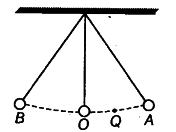# Why does a saw become warm when it is used to cut a log of wood?

(i) Illustrate the law of conservation of energy by discussing the energy changes which occur when we draw a pendulum bob to one side and allow it to oscillate.
(ii) Why does a saw become warm when it is used to cut a log of wood?

(i)shown in figure above, simple pendulum consists of a spherical metal bob suspended by a thread from a fixed support.
When the bob is displaced to end A, it gains potential energy (= mgh), here KE is zero. As it is released from rest, its potential energy begins to decrease and kinetic energy begins to increase. At an intermediate position, say point Q, the energy is partly kinetic and partly potential. At mean position, say O, the energy is totally kinetic, here PE is zero.
At the other end B, again the energy becomes totally potential, here KE is zero. Therefore, at all points, total mechanical energy remains constant which is equal to mgh.
This is the law of conservation of energy. After sometime, the energy of the bob gradually decreases as it is used in doing work against the force of friction at the point of suspension and also against friction of air, This spent energy is converted into heat. Due to decrease in the energy of the bob, its amplitude of oscillation decreases with time and eventually the bob comes to rest.
(ii) When saw is used to cut a log of wood, work is done against the force of friction between the saw and log, therefore the work done (mechanical energy) is converted into heat energy. So, it becomes warm.